# Derived functor

(diff) ← Older revision | Latest revision (diff) | Newer revision → (diff)

A functor "measuring" the deviation of a given functor from being exact. Letbe an additive functor from the product of the category of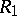-modules with the category of-modules into the category of-modules that is covariant in the first argument and contravariant in the second argument. From an injective resolutionofand a projective resolutionofone obtains a doubly-graded complex. The homology of the associated single complexdoes not depend on the choice of resolutions, has functorial properties and is called the right derived functor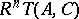of. The basic property of a derived functor is the existence of long exact sequences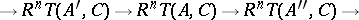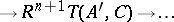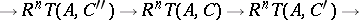induced by short exact sequences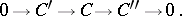The left derived functor is defined analogously. The derived functor of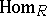is denoted by. The group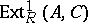classifies extensions ofwith kernelup to equivalence (cf. Baer multiplication; Cohomology of algebras).

How to Cite This Entry:
Derived functor. Encyclopedia of Mathematics. URL: http://encyclopediaofmath.org/index.php?title=Derived_functor&oldid=15566
This article was adapted from an original article by V.E. Govorov (originator), which appeared in Encyclopedia of Mathematics - ISBN 1402006098. See original article